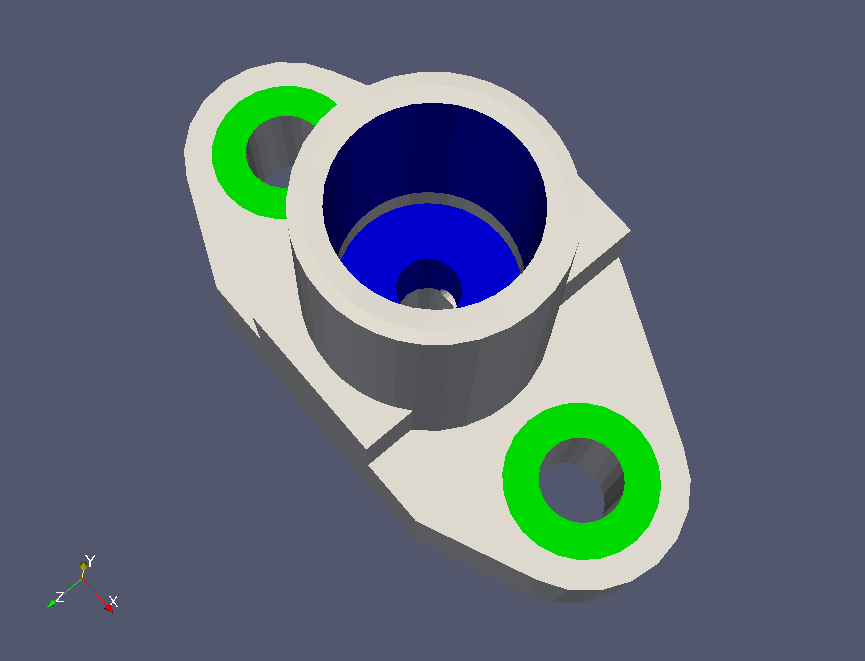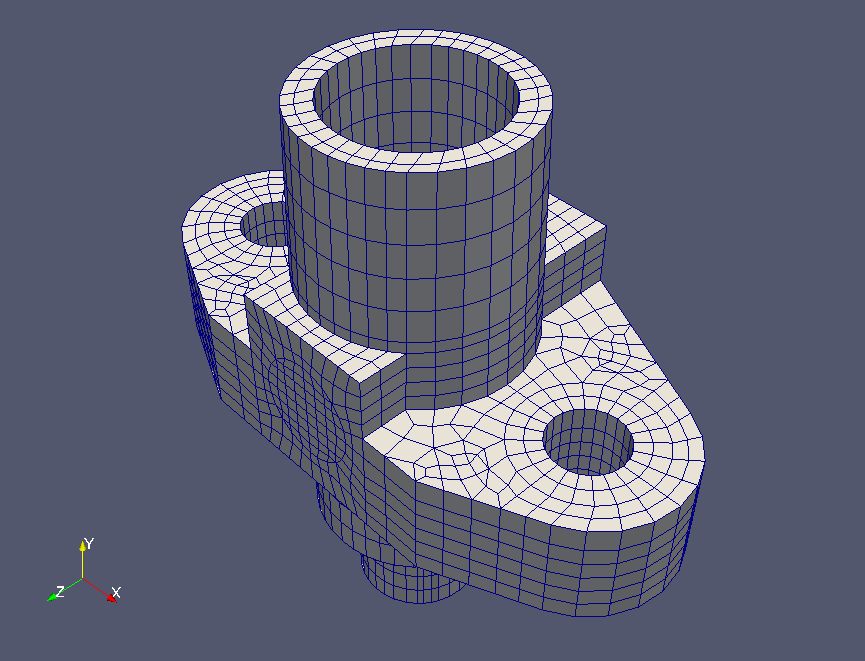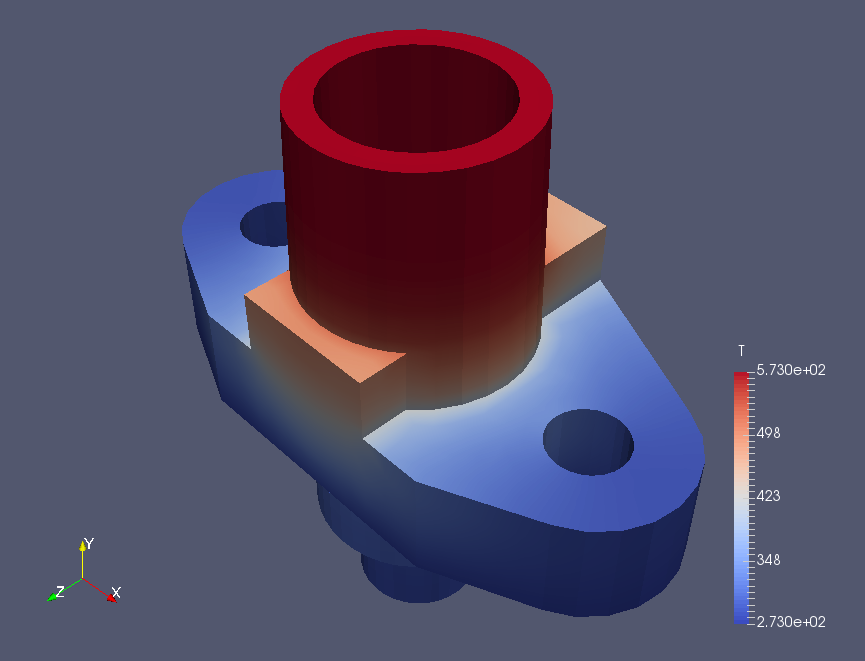﻿ Heat conduction analysis of flange - XSim

# Heat conduction analysis of flange

Update: June 1, 2017
OpenFOAM 4.x

$FOAM_TUTORIALS/basic/laplacianFoam/flange ## Summary Heat conduction equationwill be solved until 3 seconds later. For boundary conditions, temperature is fixed at 273 K for patch2 (red part) and 573 K for patch4 (blue part). All other boundaries are set to zero gradient.Boundary region (lower)Boundary region (upper) The meshes are as follows.Meshes The calculation result is as follows. Temperature distribution (T)Temperature distribution at 3 seconds (T) From the calculation results, we can see how the temperature is conducted. ## Commands cp -r$FOAM_TUTORIALS/basic/laplacianFoam/flange flange
cd flange

ansysToFoam flange.ans -scale 0.001

laplacianFoam

foamToEnsight
foamToEnsightParts
foamToVTK

paraFoam --data=VTK/flange_..vtk

The meshes of the pre-prepared ANSYS format file are converted and used. After the calculation, the result data is converted to VTK format and visualized.

## Calculation time

3.8 seconds *Single, Inter(R) Core(TM) i7-2600 CPU @ 3.40GHz 3.40GHz